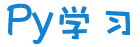注册    登录创作新主题

Python
 python开源   Django   Python   DjangoApp   pycharm
DATA
 docker   Elasticsearch

 问与答   闲聊   招聘   翻译   创业   分享发现   分享创造   求职   区块链   支付之战
aigc
 aigc   chatgpt
WEB开发
 linux   MongoDB   Redis   DATABASE   NGINX   其他Web框架   web工具   zookeeper   tornado   NoSql   Bootstrap   js   peewee   Git   bottle   IE   MQ   Jquery

Python88.com
 反馈   公告   社区推广

Py学习  »  机器学习算法

# Determinantal Point Process：机器学习中行列式的妙用

PaperWeekly • 4 月前 • 43 次点击©PaperWeekly 原创 · 作者 | Yunpeng Tai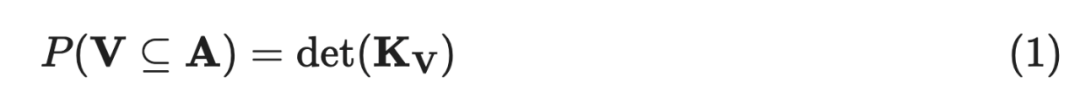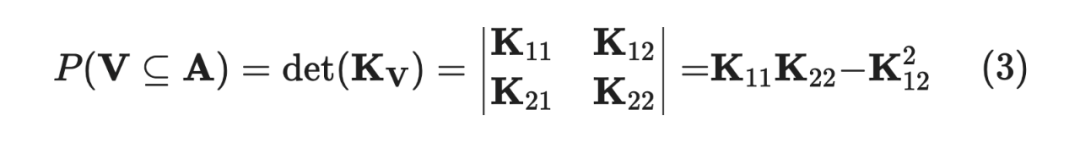L-Ensemble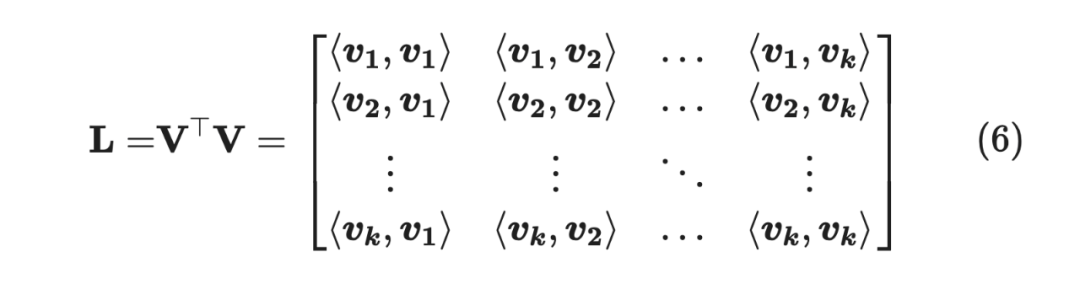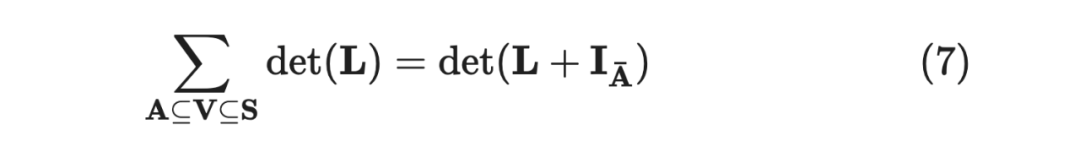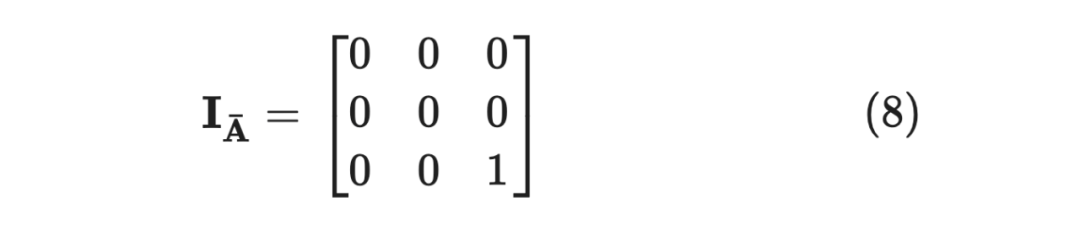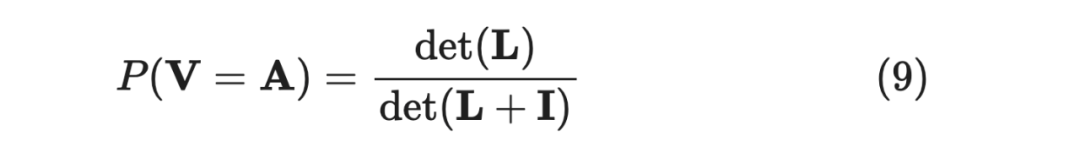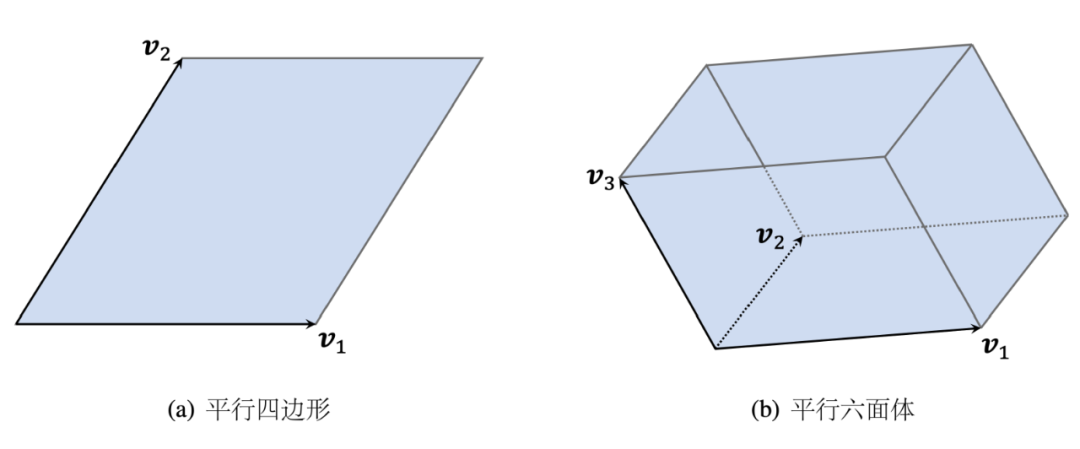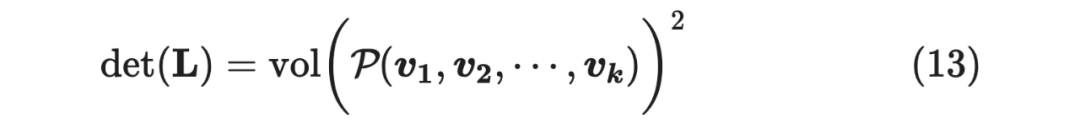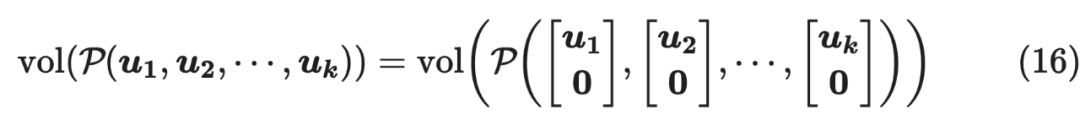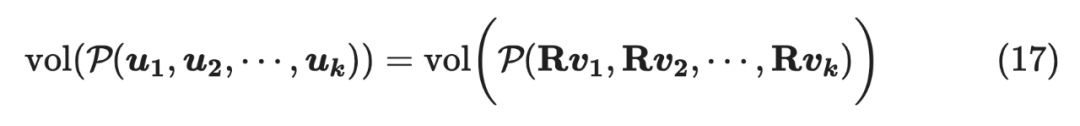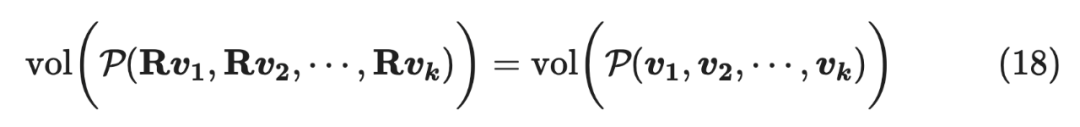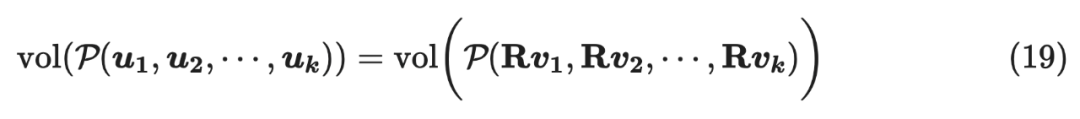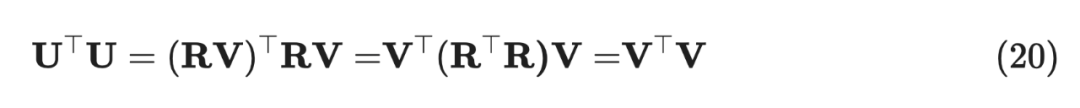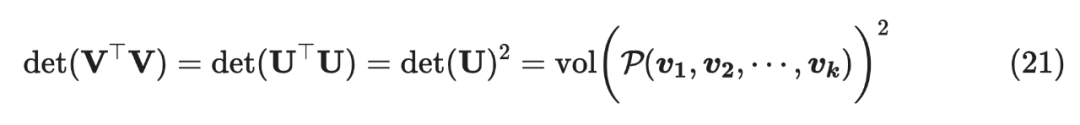Demo

``````from torch import det, eyefrom transformers import set_seedfrom transformers import BertModel, BertTokenizerset_seed(42)pretrain_path = "fabriceyhc/bert-base-uncased-imdb"model = BertModel.from_pretrained(pretrain_path).cuda()tk = BertTokenizer.from_pretrained(pretrain_path)input_text = [    "I am happy because the weather is extremely good!",    "I hate the bad weather",    "The weather is extremely good!",]inputs = tk(input_text, max_length=128, return_tensors="pt", truncation=True, padding=True)inputs = {k: v.cuda() for k, v in inputs.items()}outputs = model(**inputs).pooler_output.Tvtv = outputs.T @ outputsgroup_12 = vtv[:2][:, [0

, 1]]I = eye(2).cuda()p_12 = det(group_12) / det(group_12 + I)group_13 = vtv[[0, 2]][:, [0, 2]]p_13 = det(group_13) / det(group_13 + I)print('采样到第一个和第二个的概率：%f'%p_12)print('采样到第一个和第三个的概率：%f'%p_13)# 采样到第一个和第二个的概率：0.983567# 采样到第一个和第三个的概率：0.923823``````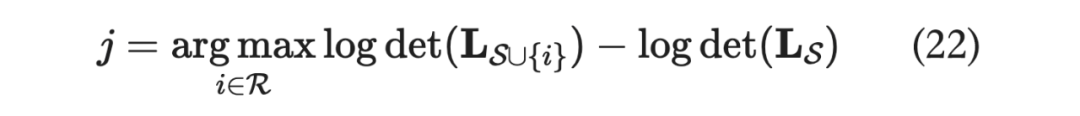Cholesky分解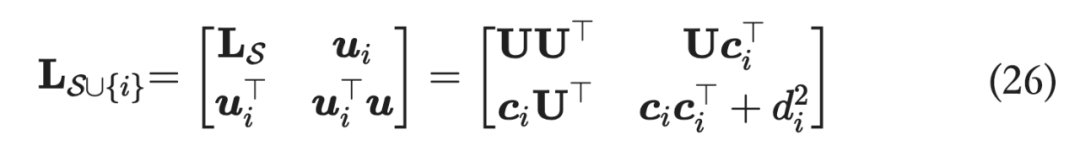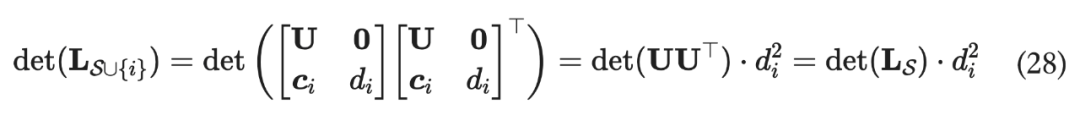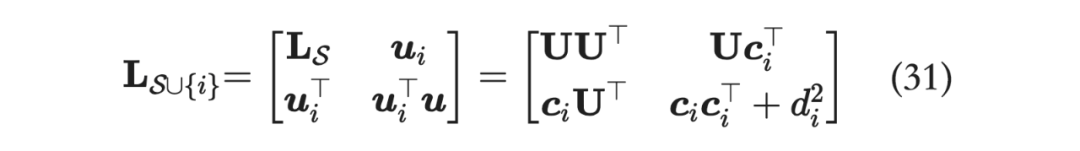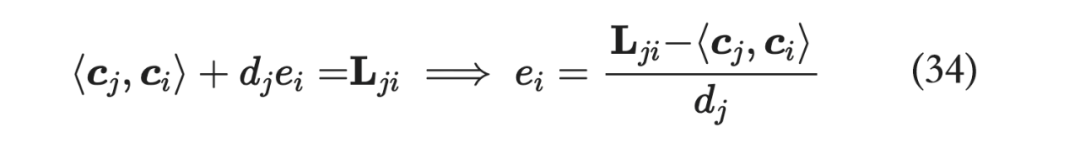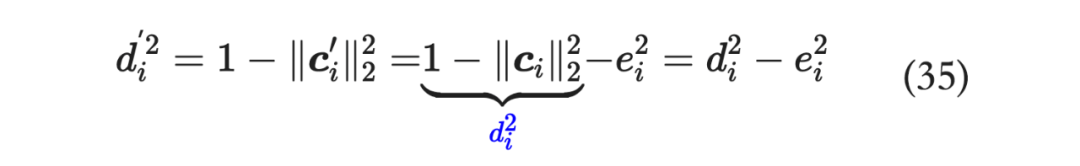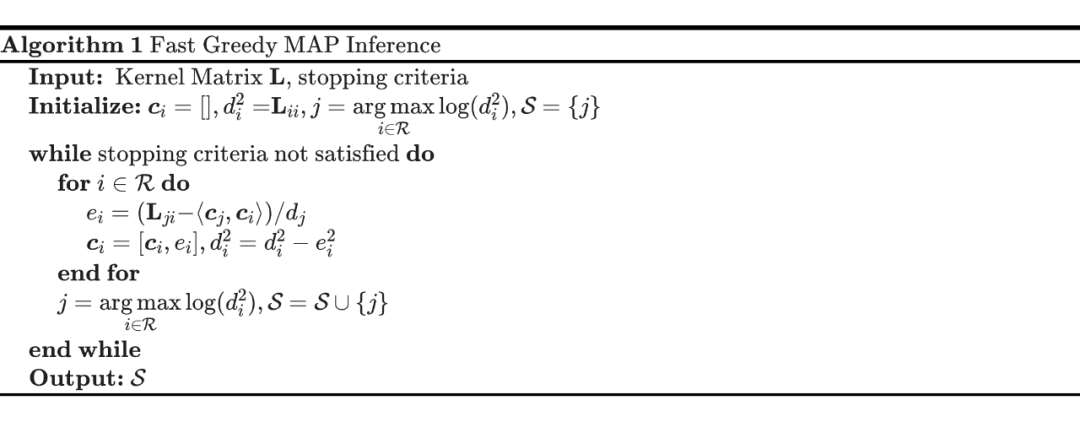``import mathimport numpy as npdef fast_map_dpp(kernel_matrix, max_length):    cis = np.zeros((max_length, kernel_matrix.shape))    di2s = np.copy(np.diag(kernel_matrix))    selected = np.argmax(di2s)    selected_items = [selected]    while len(selected_items)         idx = len(selected_items) - 1        ci_optimal = cis[:idx, selected]        di_optimal = math.sqrt(di2s[selected])        elements = kernel_matrix[selected, :]        eis = (elements - ci_optimal @ cis[:idx, :]) / di_optimal        cis[idx, :] = eis        di2s -= np.square(eis)        di2s[selected] = -np.inf        selected = np.argmax(di2s)        selected_items.append(selected)    return selected_items``

```

`原句：我现在申请微粒货？['我现在申请微粒货？', '申请微贷粒', '申请微贷粒', '我想申请微粒贷', '可以么想申请微粒贷', '微粒貸申请', '微粒貸申请', '如何申请微粒', '我现在需要申请', '我可以申请微粒贷吗', '怎么申请微粒货', '微粒貸申请', '如何申请微粒', '我可以申请微粒贷吗', '什么情况下才能申请微粒', '我要求申请', '开通微粒货', '开通微粒貨', '开通微粒货', '可以申请开通吗', '开通微粒货', '开通微粒货', '怎么申请微粒货', '申请贷款', '如何申请微粒贷', '怎么申请微粒货', '开通微粒貨', '如何申请微粒', '想办理微粒贷业务', '申请贷款', '可以申请开通吗', '我要微粒贷', '我要微粒贷', '可以么想申请微粒贷', '开通微米粒', '想开通', '我要微粒贷', '如何申请微粒', '想开通', '开通微粒貨', '开通粒微贷', '何时才能申请啊', '现在想获取资格', '怎么申请微粒货', '开通申请', '开通', '开通', '你好我申请借款', '开通微']````

``['我现在申请微粒货？', '开通', '何时才能申请啊', '怎么申请微粒货', '你好我申请借款', '现在想获取资格', '我可以申请微粒贷吗', '我要微粒贷', '微粒貸申请', '什么情况下才能申请微粒']``Sliding Window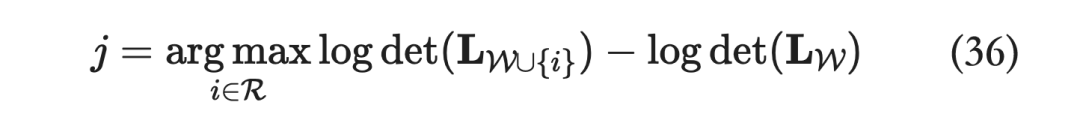Window size 的选择也是比较重要的，不妨看一些 demo：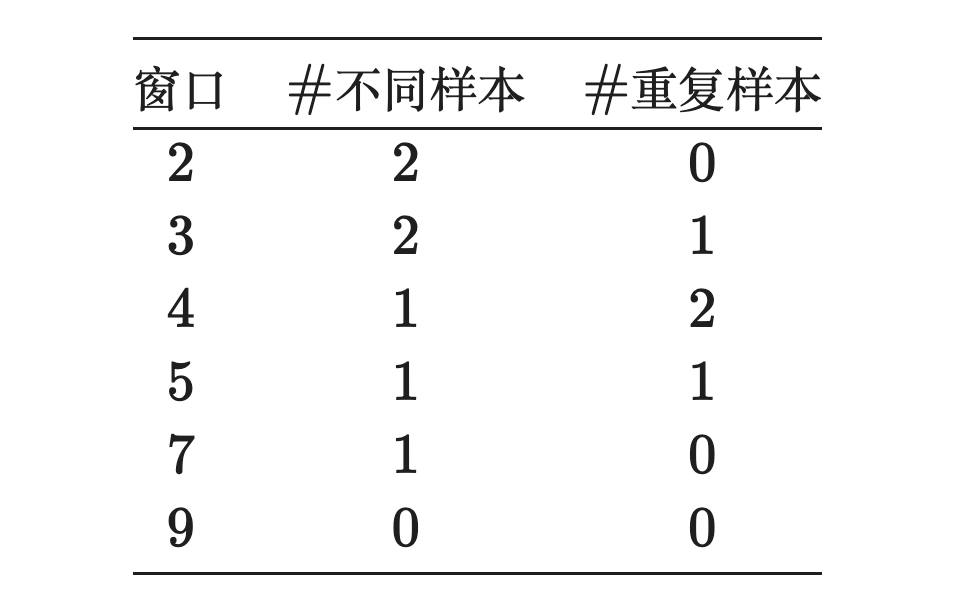``w/o window['开通', '怎么申请微粒货', '何时才能申请啊', '现在想获取资格', '我要微粒贷', '微粒貸申请', '我可以申请微粒贷吗', '什么情况下才能申请微粒', '你好我申请借款', '我现在申请微粒货？']``
``w/ window['开通', '开通申请', '怎么申请微粒货', '何时才能申请啊', '现在想获取资格', '我要微粒贷', '我可以申请微粒贷吗', '可以申请开通吗', '如何申请微粒', '我现在申请微粒货？']``#投 稿 通 道#

让你的文字被更多人看到

PaperWeekly 鼓励高校实验室或个人，在我们的平台上分享各类优质内容，可以是最新论文解读，也可以是学术热点剖析科研心得竞赛经验讲解等。我们的目的只有一个，让知识真正流动起来。

📝 稿件基本要求：

• 文章确系个人原创作品，未曾在公开渠道发表，如为其他平台已发表或待发表的文章，请明确标注

• 稿件建议以 markdown 格式撰写，文中配图以附件形式发送，要求图片清晰，无版权问题

• PaperWeekly 尊重原作者署名权，并将为每篇被采纳的原创首发稿件，提供业内具有竞争力稿酬，具体依据文章阅读量和文章质量阶梯制结算

📬 投稿通道：

• 投稿邮箱：hr@paperweekly.site

• 来稿请备注即时联系方式（微信），以便我们在稿件选用的第一时间联系作者

• 您也可以直接添加小编微信（pwbot02）快速投稿，备注：姓名-投稿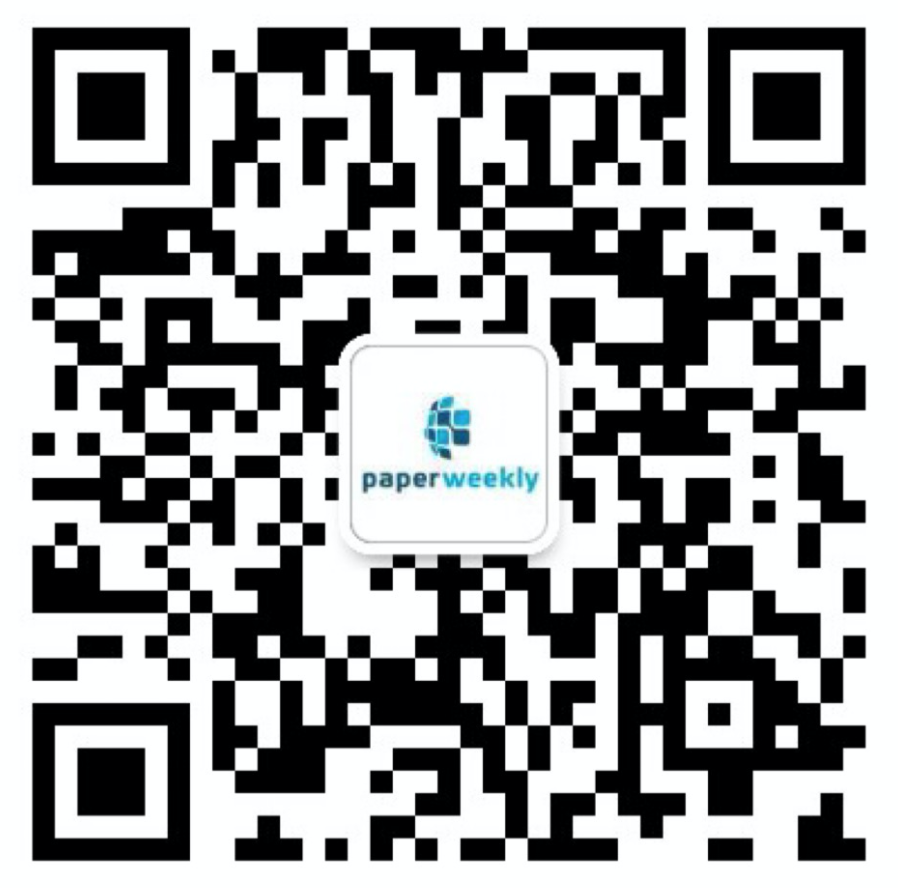△长按添加PaperWeekly小编

🔍

·
·Python社区是高质量的Python/Django开发社区